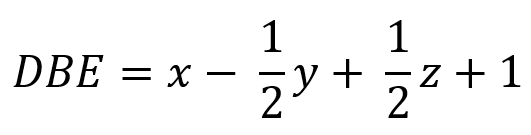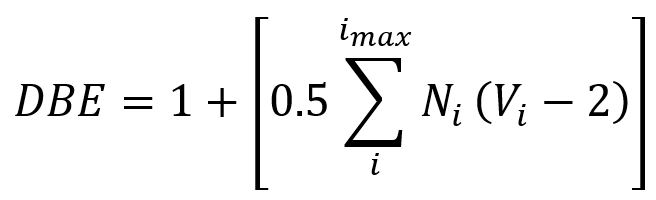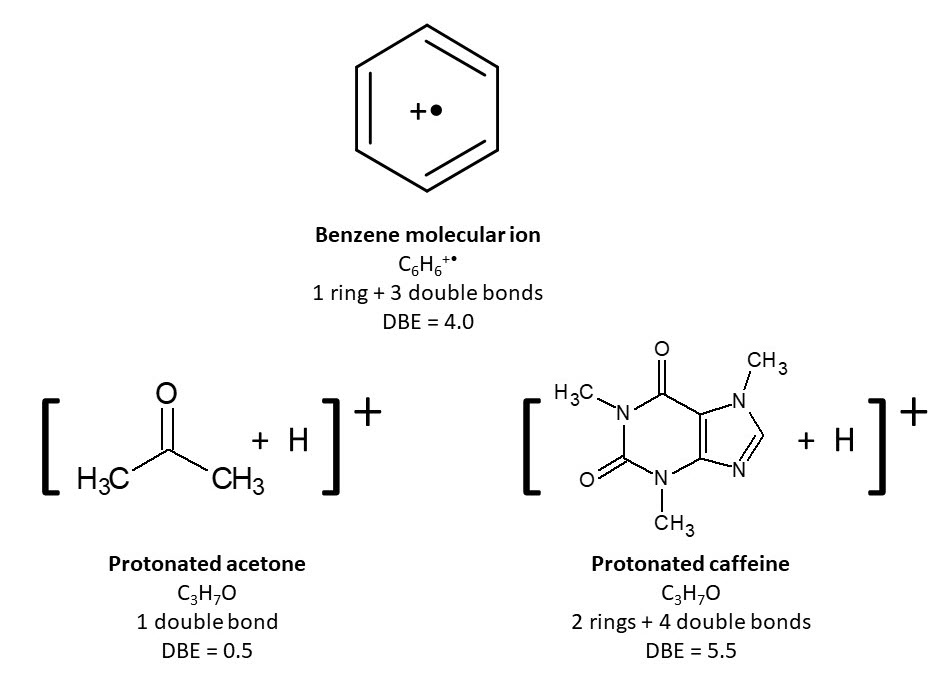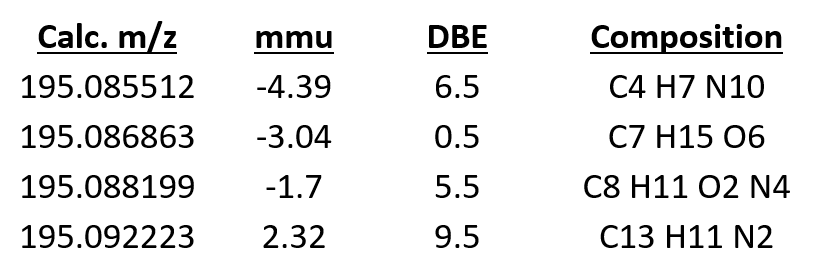# A deeper dive into elemental composition determination with DART

Learn how constraints limit the possible elemental compositions for DART-MS with accurate mass information.

In the last article, we found 8 possible elemental compositions for a protonated molecule having a positive-ion DART-MS peak at m/z 195.0899 +/- 0.005 and elements C, H, N, and/or O. We can use constraints such as unsaturation (double bond equivalents) to eliminate many of the incorrect compositions.

## Electron configuration

Ions in a mass spectrum fall into two categories: even-electron ions and odd-electron ions (radical ions). Odd-electron ions have an unpaired electron. An example of an odd-electron ion is the molecular ion M+• in electron ionization (EI) mass spectra. Protonated molecules [M + H]+ that are formed in positive-ion DART-MS are even-electron ions. DART-MS produces primarily even-electron ions1. Even-electron ions are also found in other mass spectra from many other ionization methods, such as chemical ionization (CI) and electrospray ionization (ESI) and as fragment ions in EI mass spectra.

#### Even-electron ions (half integer unsaturation):

• Protonated molecule [M+H]+
• Deprotonated molecule [M+H]-
• Ammoniated molecule [M+NH4]+
• Fragment F+

#### Even-electron ions (exact integer unsaturation):

• Fragment F+
Examples of even-electron and odd-electron ions

## Double bond equivalents

For any given elemental composition, we can calculate a number called Double Bond Equivalents (DBE, or unsaturation) that represents the number of rings and sites of unsaturation (double and triple bonds) that would occur in molecules with the given composition. This is calculated using a formula based on the number and valence of each element in the composition. For compounds with a composition CxHyNzOn, the formula is:The more general formula2 is:where DBE is the unsaturation, imax is the total number of different elements in the composition, Ni the number of atoms of element i, and Vi is the valence of atom i.

If we have additional information about the unknown molecule, such as NMR or FTIR data, we might be able to set limits on the range of DBE values for our unknown.

## Using the DBE value to distinguish even-electron and odd-electron ions

Assuming that we don’t have additional information, why is this value is still useful? Simple! The calculated double bond equivalents for odd-electron ions are whole integers, but the calculated double bond equivalents for even-electron ions are half-integer values.## Application to our accurate-mass DART-MS example

Our elemental composition software calculates a DBE for each candidate composition determined from the accurate-mass data. Because we are using DART-MS, an ionization method such as that produces primarily even-electron ions, we can display only compositions with a half-integer DBE to eliminate all but four compositions for our peak at m/z 195.0899.Constraining our elemental compositions to even-electron ions eliminated half of the compositions. We happen to know that the correct composition is in this list, but in practice we would have to choose between the four remaining compositions. The next article in this series will explain how to use isotopic data to rank the compositions.
If you know what kind of ions are formed by an ionization method such as DART-MS, you may be able to eliminate many incorrect elemental compositions determined from accurate-mass spectra. You can read more about determining elemental compositions here. To learn more about JEOL mass spectrometers and the AccuTOF-DART system, please visit us here.

### Notes

1Odd-electron ions are rare in DART-MS, but they can occur in certain circumstances. This will be the subject of a future post

2This formula is only going to give the correct number of double bond equivalents for elements in their lowest valence state. Be careful when you are interpreting DBE values for compositions with elements such as S and P that have more than one common valence state.

## News & Events Quick LinksJEOL USA, Inc.